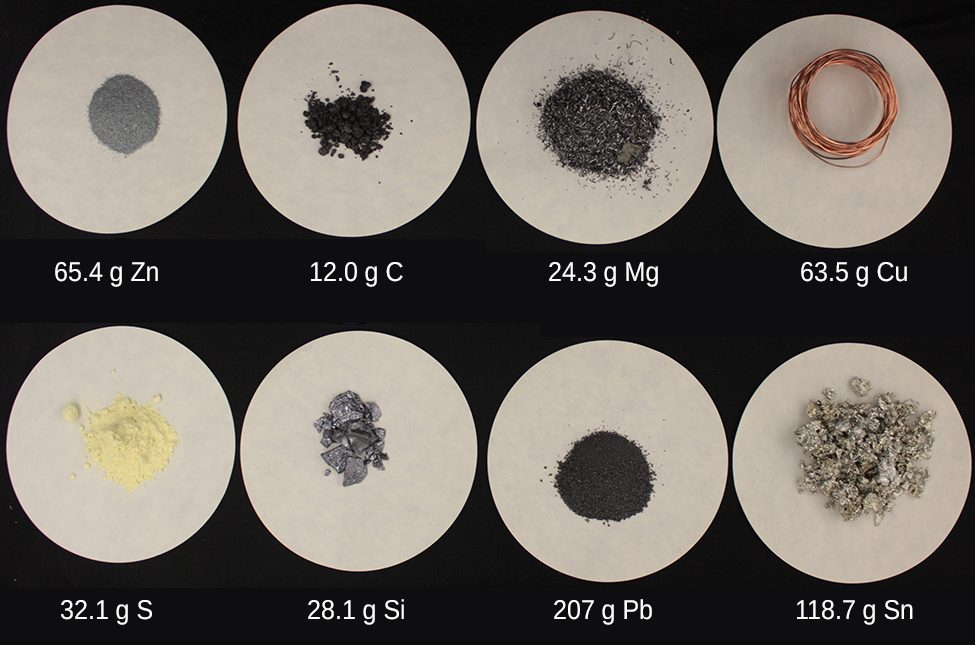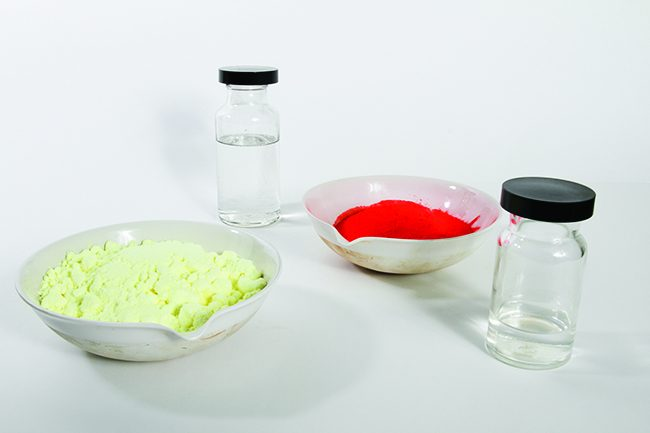# The mole concept

• Particles are classified as either: Atoms, ions, molecules or formula units
• To perform chemistry, moles of substance are used, and this allows us to make comparisons between chemical species.

### The Mole

• Avogadro’s constant N= 6.02 x 1023 mol-1
• Mole: a fixed number of particles and refers to the amount, n, of substance
• Molar mass: mass of 1 mole of a substance (g mol-1)
• Number prefixes which are important to know:
•### Mole Calculations### Relative atomic mass & molar mass

• Isotopes: Atoms of the same element which have same number of protons
• Isotopes of an element have different mass numbers
• Relative abundance: Measure of percentage of isotopes present in element
• Relative atomic mass (Ar): weighted average of the atomic masses of its isotopes and their relative abundances
• Relative because compared to 1 atom of carbon-12 (12C) which is 12 units
• Relative molecular mass (Mr): Combining individuals Ar values of atoms in molecule or formula unit

### Empirical and molecular formula determination

• Empirical formula: simplest whole number ratio of atoms or amount (in mol) of each element present in a compound
• Molecular Formula: the actual number of atoms or amount (in mol) of elements in one structural unit or one mole of the compound
• The identity of a substance is defined not only by the types of atoms or ions it contains, but by the quantity of each type of atom or ion. For example, water, H2O, and hydrogen peroxide, H2O2, are alike in that their respective molecules are composed of hydrogen and oxygen atoms.
• However, because a hydrogen peroxide molecule contains two oxygen atoms, as opposed to the water molecule, which has only one, the two substances exhibit very different properties.
• Today, we possess sophisticated instruments that allow the direct measurement of these defining microscopic traits; however, the same traits were originally derived from the measurement of macroscopic properties (the masses and volumes of bulk quantities of matter) using relatively simple tools (balances and volumetric glassware).
• This experimental approach required the introduction of a new unit for amount of substances, the mole, which remains indispensable in modern chemical science.
• The mole is an amount unit similar to familiar units like pair, dozen, gross, etc. It provides a specific measure of the number of atoms or molecules in a bulk sample of matter.

A mole is defined as the amount of substance containing the same number of discrete entities (such as atoms, molecules, and ions) as the number of atoms in a sample of pure 12C weighing exactly 12 g.

• One Latin connotation for the word “mole” is “large mass” or “bulk,” which is consistent with its use as the name for this unit.
• The mole provides a link between an easily measured macroscopic property, bulk mass, and an extremely important fundamental property, number of atoms, molecules, and so forth.
• The number of entities composing a mole has been experimentally determined to be 6.02214179 × 1023, a fundamental constant named Avogadro’s number (NA) or the Avogadro constant in honor of Italian scientist Amedeo Avogadro.
• This constant is properly reported with an explicit unit of “per mole,” a conveniently rounded version being 6.022 × 1023/mol.
• Consistent with its definition as an amount unit, 1 mole of any element contains the same number of atoms as 1 mole of any other element.
• The masses of 1 mole of different elements, however, are different, since the masses of the individual atoms are drastically different.
• The molar mass of an element (or compound) is the mass in grams of 1 mole of that substance, a property expressed in units of grams per mole (g/mol)Each sample contains 6.022 × 1023 atoms —1.00 mol of atoms. From left to right (top row): 65.4 g zinc, 12.0 g carbon, 24.3 g magnesium, and 63.5 g copper. From left to right (bottom row): 32.1 g sulfur, 28.1 g silicon, 207 g lead, and 118.7 g tin. (credit: modification of work by Mark Ott)
• Because the definitions of both the mole and the atomic mass unit are based on the same reference substance, 12C, the molar mass of any substance is numerically equivalent to its atomic or formula weight in amu.
• Per the amu definition, a single 12C atom weighs 12 amu (its atomic mass is 12 amu). According to the definition of the mole, 12 g of 12C contains 1 mole of 12C atoms (its molar mass is 12 g/mol).
• This relationship holds for all elements, since their atomic masses are measured relative to that of the amu-reference substance, 12C. Extending this principle, the molar mass of a compound in grams is likewise numerically equivalent to its formula mass in amuEach sample contains 6.02 × 1023 molecules or formula units—1.00 mol of the compound or element. Clock-wise from the upper left: 130.2 g of C8H17OH (1-octanol, formula mass 130.2 amu), 454.4 g of HgI2(mercury(II) iodide, formula mass 454.4 amu), 32.0 g of CH3OH (methanol, formula mass 32.0 amu) and 256.5 g of S8 (sulfur, formula mass 256.5 amu)
Element Average Atomic Mass (amu) Molar Mass (g/mol) Atoms/Mole
C 12.01 12.01 6.022 × 1023
H 1.008 1.008 6.022 × 1023
O 16.00 16.00 6.022 × 1023
Na 22.99 22.99 6.022 × 1023
Cl 35.45 33.45 6.022 × 1023

While atomic mass and molar mass are numerically equivalent, keep in mind that they are vastly different in terms of scale, as represented by the vast difference in the magnitudes of their respective units (amu versus g).

To appreciate the enormity of the mole, consider a small drop of water weighing about 0.03 g. Although this represents just a tiny fraction of 1 mole of water (~18 g), it contains more water molecules than can be clearly imagined.

If the molecules were distributed equally among the roughly seven billion people on earth, each person would receive more than 100 billion molecules.The number of molecules in a single droplet of water is roughly 100 billion times greater than the number of people on earth
• The relationships between formula mass, the mole, and Avogadro’s number can be applied to compute various quantities that describe the composition of substances and compounds.
• For example, if we know the mass and chemical composition of a substance, we can determine the number of moles and calculate number of atoms or molecules in the sample.
• Likewise, if we know the number of moles of a substance, we can derive the number of atoms or molecules and calculate the substance’s mass.

UNDERSTANDING THE MOLE CONCEPT

## STEM Elearning

We at FAWE have built this platform to aid learners, trainers and mentors get practical help with content, an interactive platform and tools to power their teaching and learning of STEM subjects, more